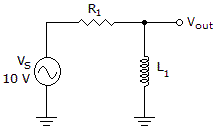# Electronics - RL Circuits - Discussion

### Discussion :: RL Circuits - General Questions (Q.No.5)

5.Which of the following statements is true if the inductor shorts out in the circuit in the given circuit?

 [A]. Each component drops 5 V. [B]. The impedance equals 0. [C]. The power factor equals 1. [D]. The phase angle equals 90°.

Explanation:

No answer description available for this question.

 Vasu said: (Oct 12, 2011) Power factor = R/Z But Z=R;as L is shorted. Hence power factor= R/R =1

 Tiieera said: (Nov 28, 2011) I completely agree with u sir..As the inductor is short circuited it behaves as a purely resistive circuit. Phase angle is zero. P.F=cos(0)=1.

 Prakash said: (Mar 21, 2013) In simple language, there will no lead or lag in the circuit, and the power factor will be 1 that the highest, or in other words ideal, that is required to save electricity bills in big companies.

 Asma Asghar Janjau said: (Jun 18, 2016) When inductor is short only resistor is present and becomes load, when load appear as resistor then power factor is 1.

 Xiyaz said: (May 21, 2019) No loss of energy, only true power consumes by resistive load so power factor is 1. No, lead or lag current or storage energy there is no reactive power. Only true power seems to convert in a proportional into heat. PF is 1.